# How to draw in two-point perspective

In this tutorial, you will learn how to draw objects in the two-point perspective (also diagonal perspective). This allows us to depict things in an oblique view in perspective.

In a previous tutorial, we got to know the one-point perspective. This technique of the perspective representation had the limitation that it could represent objects only frontally from the front perspective. However, the two-point perspective - also often referred to as a perspective with two vanishing points - represents objects that are positioned obliquely to the observer. You can learn how to draw in two-point perspective in the following exercise.

In the following picture you see several cuboids in the two-point perspective*, with the worm’s-eye view, the normal perspective and the bird’s-eye view being shown.

## Exercise: Draw a cuboid in the two-point perspective

In the following exercise we will try to draw a simple cuboid in the two-point perspective. The cuboid is one of the simplest motifs for this technique.
The procedure will be explained step by step so that beginners of the perspective drawing can easily understand the whole procedure.

### Step 1 - Basic elements of the two-point perspective

In the two-point perspective you need two vanishing points. Therefore, draw the horizon first and mark two vanishing points - left and right.### Step 2 - Vanishing Lines

Starting from the two vanishing points, two vanishing lines are defined, each defining the underside of the cuboid.### Step 3 - Draw the lower surface

The bottom of the cuboid can now be drawn using the vanishing lines.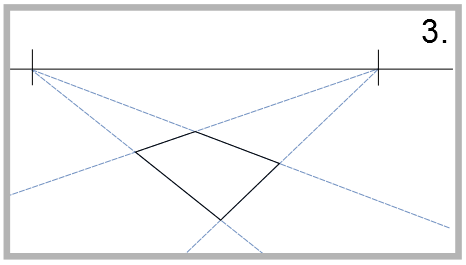### Step 4 - Set the height of the cuboid

Next, the foremost edge of the cuboid follows. This edge defines the height of the cuboid.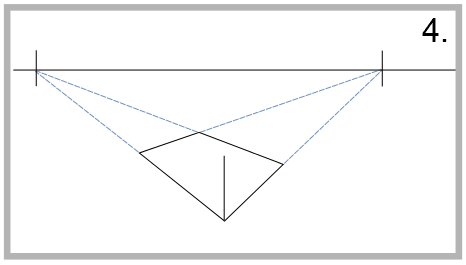### Step 5 - Prepare top surface

Now draw two lines from the left and right vanishing point to the top of the edge. This is used to prepare the geometry of the upper surface of the cuboid.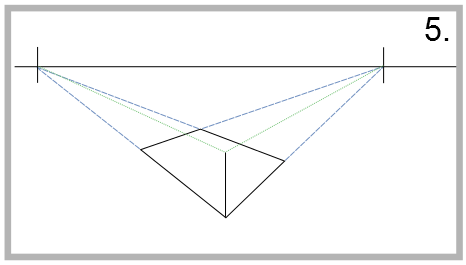### Step 6 - Draw side edges

With the help of these vanishing lines, the two lateral edges can now be raised vertically. The edges end at the two new vanishing lines. In the two-point perspective, these two lines are automatically shorter than the front edge, as the condition of the perspective mode of presentation requires.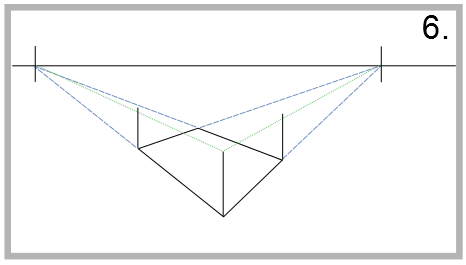### Step 7 - Vanishing lines of the rear upper edges

To the points at which these two lateral edges intersect the upper lines, two further lines can be drawn. These vanishing lines mark the two rear upper edges of the cuboid.

Drawing in one-point perspective

### Step 8 - Draw the rear vertical edge

The two new escape lines define the height of the rear vertical edge of the cuboid.### Step 9 - Define the upper surface

The form of the surface of the cuboid is also defined with the preceding step. Now you can trace these lines clearly with the pencil.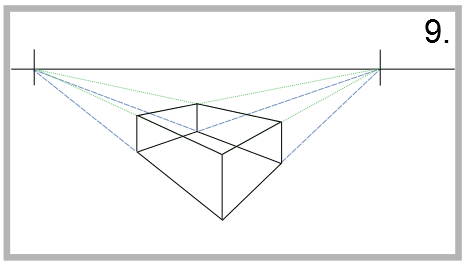### Step 10 - Remove the guide lines

Now you can erase all the auxiliary lines and the hidden edges. Thus, the perspective drawing of the cuboid is completed in the two-point perspective.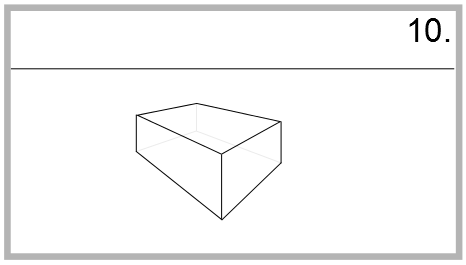After this last step, the drawing is finished. This exercise is, of course, a very simple example of the two-point perspective. However, you should have learned how the perspective works with two vanishing points and now be able to implement your own simple perspective drawings with this method.